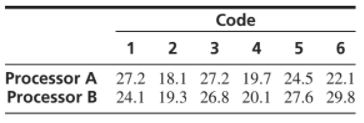×
Get Full Access to Statistics For Engineers And Scientists - 4 Edition - Chapter 6.8 - Problem 6e
Get Full Access to Statistics For Engineers And Scientists - 4 Edition - Chapter 6.8 - Problem 6e

×

# Two microprocessors are compared on a sample of sixISBN: 9780073401331 38

## Solution for problem 6E Chapter 6.8

Statistics for Engineers and Scientists | 4th Edition

• Textbook Solutions
• 2901 Step-by-step solutions solved by professors and subject experts
• Get 24/7 help from StudySoup virtual teaching assistantsStatistics for Engineers and Scientists | 4th Edition

4 5 1 285 Reviews
25
4
Problem 6E

Two microprocessors are compared on a sample of six benchmark codes to determine whether there is a difference in speed. The times (in seconds) used by each processor on each code are given in the following table.Can you conclude that the mean speeds of the two processors differ?

Step-by-Step Solution:

Step 2 of 10

Step 3 of 10

##### ISBN: 9780073401331

The full step-by-step solution to problem: 6E from chapter: 6.8 was answered by , our top Statistics solution expert on 06/28/17, 11:15AM. Since the solution to 6E from 6.8 chapter was answered, more than 451 students have viewed the full step-by-step answer. Statistics for Engineers and Scientists was written by and is associated to the ISBN: 9780073401331. This textbook survival guide was created for the textbook: Statistics for Engineers and Scientists , edition: 4. This full solution covers the following key subjects: Microprocessors, Benchmark, Codes, compared, conclude. This expansive textbook survival guide covers 153 chapters, and 2440 solutions. The answer to “?Two microprocessors are compared on a sample of six benchmark codes to determine whether there is a difference in speed. The times (in seconds) used by each processor on each code are given in the following table. Can you conclude that the mean speeds of the two processors differ?” is broken down into a number of easy to follow steps, and 49 words.

## Discover and learn what students are asking

Calculus: Early Transcendental Functions : Surface Integrals
?In Exercises 1 - 4, evaluate $$\int_{S} \int(x-2 y+z) d S$$. $$S: z=2, x^{2}+y^{2} \leq 1$$

Statistics: Informed Decisions Using Data : Organizing Qualitative Data
?What is a bar graph? What is a Pareto chart?

Statistics: Informed Decisions Using Data : Testing the Significance of the Least-Squares Regression Model
?In the least-squares regression model, yi = b1xi + b0 + ei, ei is a random error term with mean ______________ and standard deviation aet = __________

Unlock Textbook Solution